Step by step Solutions and Answers to Operations Research – Modeling with Linear Programming and Minimization Models. The solutions are done using the R programming language along with the diagrams and graphs. To view my code that I did on the R programming language, open my github page https://github.com/jamesadhitthana/UPH_OR_2018

ENJOY!

# Set 2.2a Question 6

Alumco manufactures aluminium sheets and aluminium bars. The maximum production capacity is estimated at either 800 sheets or 600 bars per day. The maximum daily demand is 550 sheets and 580 bars . The profit per ton is \$40 per sheet and \$35 per bar. Determine the the optimal daily production mix.

X1 = sheets

X2 = bars

Maximize

Z = 40X1 + 35X2

In constraint to

1/800X1 + 1/600X2 <= 1

X1 <= 550

X2 <= 580

Result:

X1 = 550

X2 = 187.5

Z = \$28562.5

Optimal daily production mix is 550 aluminum sheets and 187.5 aluminum bars to get a profit of \$28562.5.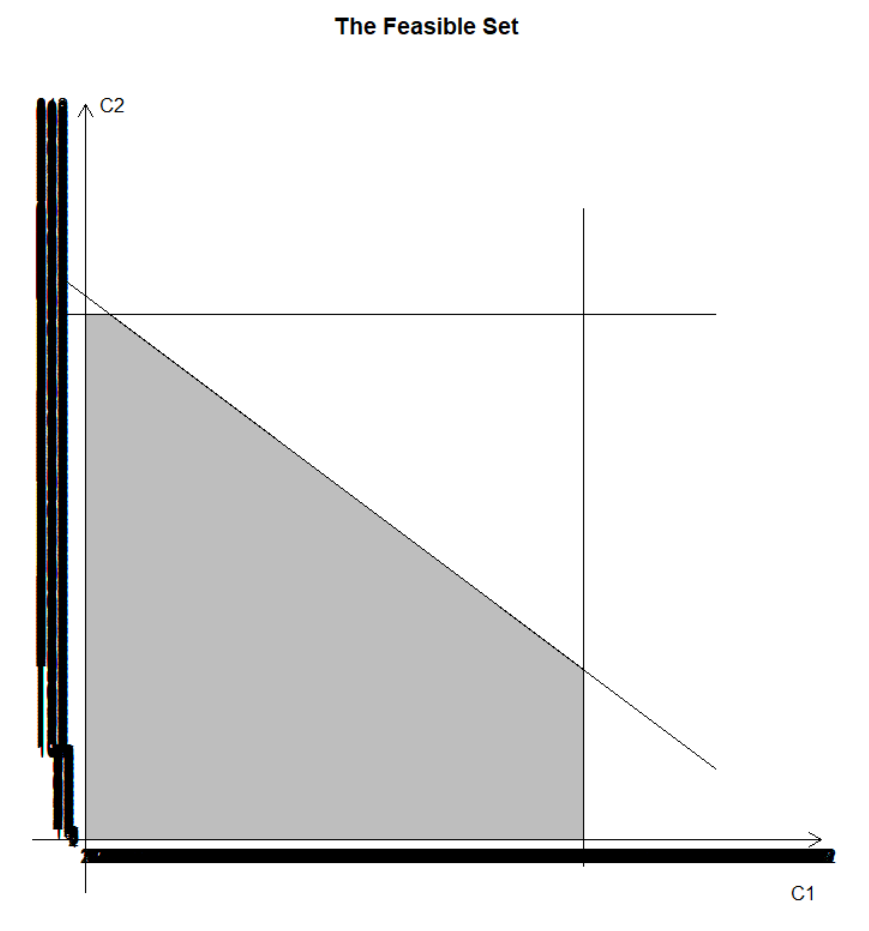# Set 2.2a – Question 9

ChemLabs uses raw materials I and II to produce two domestic cleaning solutions, A and B. The daily availabilities of raw materials I and II are 150 and 145 units, respectively. One unit of solution A consumes .5 unit of raw material I and .6 unit of raw material II, and one unit of solution B uses .5 unit of raw material I and .4 unit of raw material II. The profits per unit of solutions A and B are \$8 and \$10, respectively. The daily demand for solution A lies between 30 and 150 units, and that for solution B between 40 and 200 units. Find the optimal production amounts of A and B.

X1 = SolutionA

X2 = SolutionB

Maximize

Z = 8X1 + 10X2

In constraint to

X1 >= 30

X1 <= 150

X2 >= 40

X2 <= 200

0.5X1 + 0.5X2 <= 150

0.6X1 + 0.4X2 <= 145

Result:

X1 = 100 units

X2 = 200 units

Z = \$2800

Optimal production for A is 100 units and B is 200 units to get a profit of \$2800.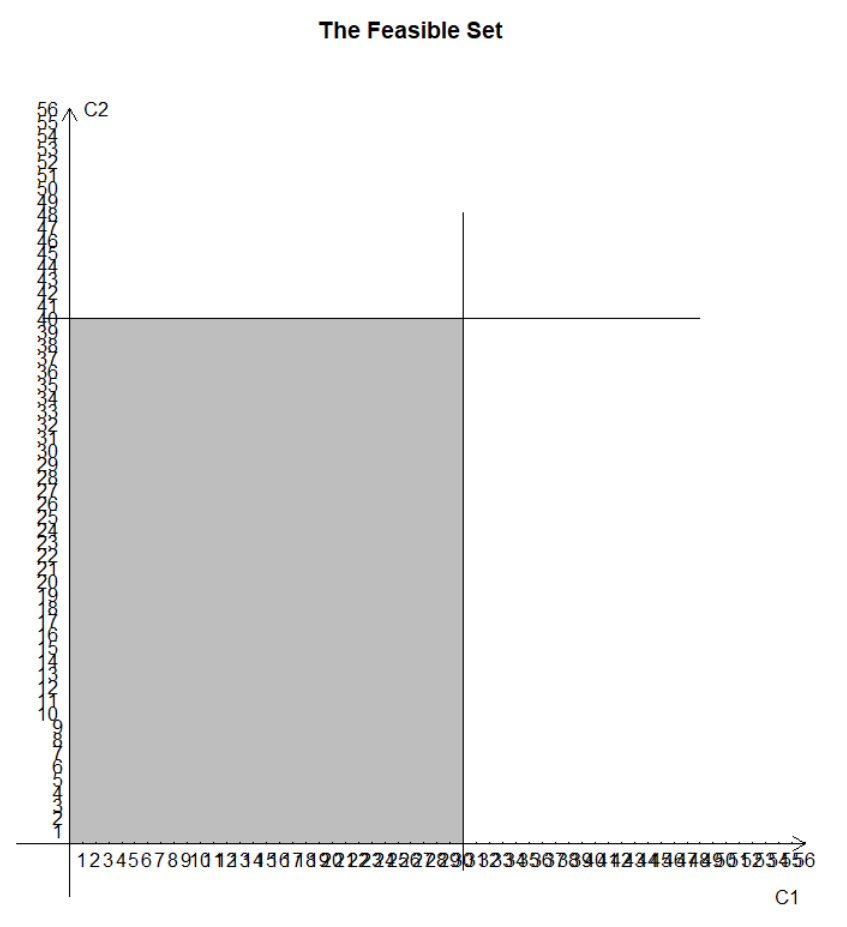# Set 2.2a – Question 12

Wild West produces two types of cowboy hats. A type 1 hat requires twice as much labor time as a type 2. If the all available labor time is dedicated to Type 2 alone, the company can produce a total of 400 Type 2 hats a day. The respective market limits for the two types are 150 and 200 hats per day. The profit is \$8 per Type 1 hat and \$5 per Type 2 hat. Determine the number of hats of each type that would maximize profit.

X1=Type1 hat

X2=Type2 hat

Maximize

Z = 8X1 + 5X2

In constraint to

2X1 + X2 <= 400

X1 <= 150

X2 <= 200

Result:

X1 = 100 hats

X2 = 200 hats

Z = \$1800

To maximize profit of \$1800 they need to make 100 Type 1 hats and 200 Type 2 hats.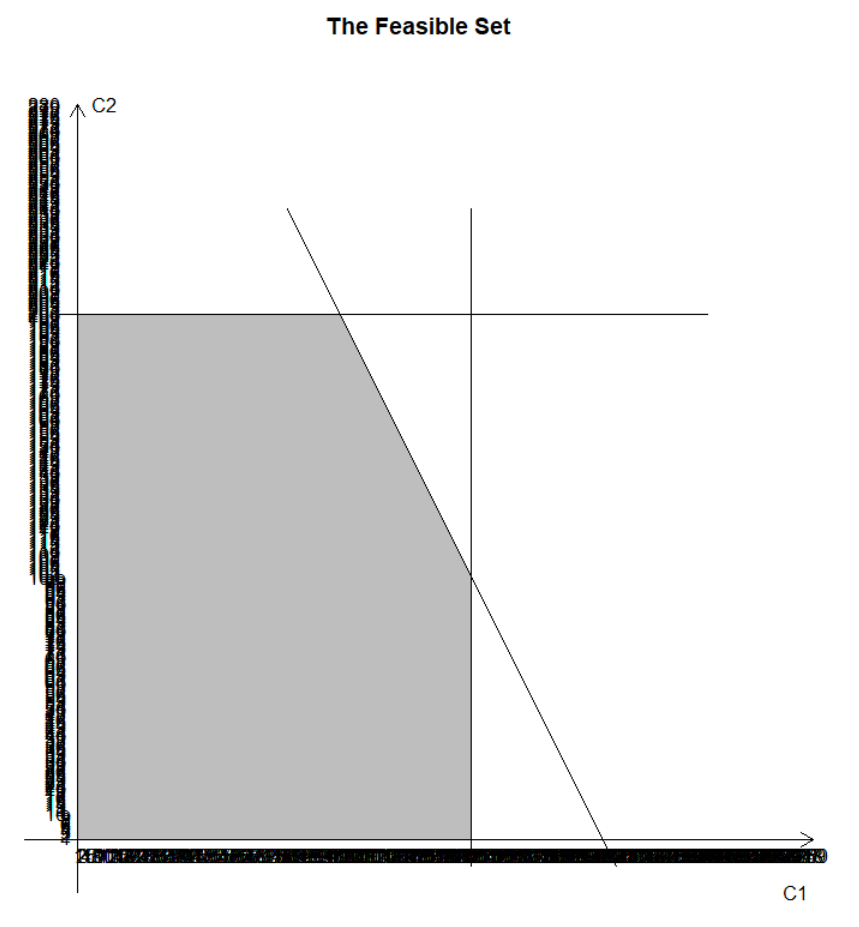# Set 2.2a – Question 13

X2=TV

Maximize

Z = X1 + 25X2

In constraint to

15X1 + 300X2 <= 10000

-X1 + 2X2 <= 0

X1 <= 400

Result:

X1 = 60.60606 minutes

X2 = 30.30303 minutes

Z = \$818.1818

Optimum Allocation is 60.60606 minutes radio time and 30.30303 minutes TV time with cost of \$818.1818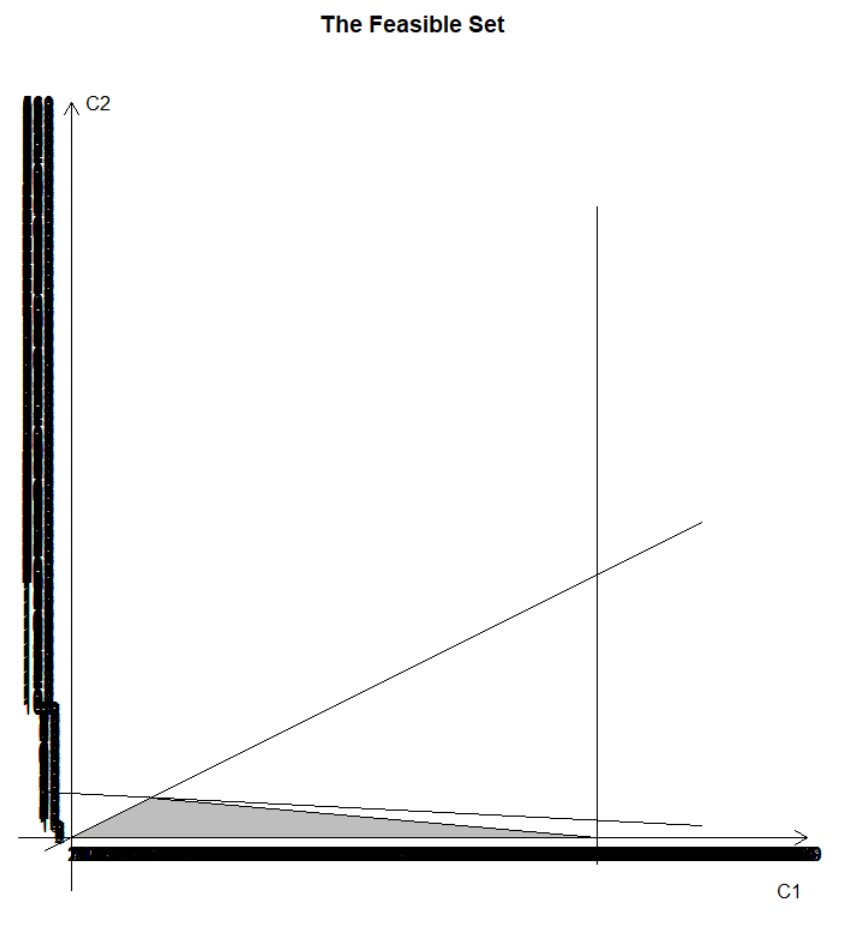# Minimization Model 2.2B – Q4

John must work at least 20 hours a week to supplement his income while attending school. He has the opportunity to work in two retail stores. In store 1, he can work between
5 and 12 hours a week, and in store 2 he is allowed between 6 and 10 hours. Both stores pay the same hourly wage. In deciding how many hours to work in each store, John wants to base his decision on work stress. Based on interviews with present employees,
John estimates that, on an ascending scale of 1 to 10, the stress factors are 8 and 6 at stores 1 and 2, respectively. Because stress mounts by the hour, he assumes that the total stress for each store at the end of the week is proportional to the number of hours he works in the store. How many hours should 10hn work in each store?

X1 = store 1 hour

X2 = store 2 hour

Minimize

Z = 8X1 + 6X2

In constraint to

X1 +X2>= 20

X1 >= 5

X1 <= 12

X2 >= 6

X2 <= 10

Result:

X1 = 10 hours

X2 = 10 hours

Z = 140 stress

John should work 10 hours in each store with a total minimum stress of 140.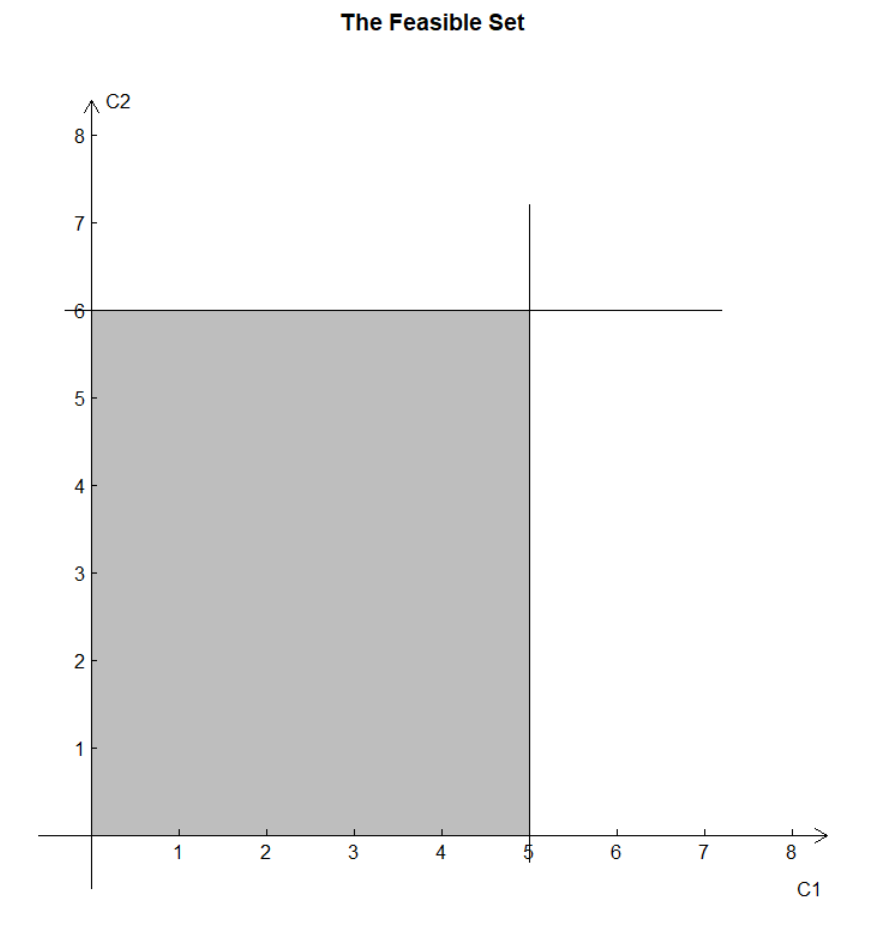#### James

Check out my instagram page! http://instagram.com/paynedeath